Home » Do While and While Loop## Do While and While Loop C/C++ Help

Do while and while loop assignment help, difference between do while and while loop.

Loop in programming is set of instructions that performs sequentially and repeated until certain condition is matched. There are different loops in programming languages for loop, do while loop and while loop.

The while loop is most basic loop of programming languages. Working of while loop define as first it can check the certain condition, The condition used in while loop is an expression of Boolean type which returns true or false.

If the condition verify means that it return true for the given condition then compiler move into the body of while loop and execute the instructions of body of loop until conditions becomes false. The body of while loop consists of instructions either a single line instruction or set of instructions to execute.

The body of while loop iterates till the given condition is true and when condition becomes false the body of while loop not execute means the while loop is terminated.

It is similar to the “for loop” of programming language which first verify the given condition and then execute the instruction of the body.

As we see that in while loop, if a certain condition becomes false in its first iteration then the body of while loop not executes. But in do-while loop the first iteration is always true and execute the body of do-while loop one time must then check the given condition.

The working functionality of do-while is define as that it first implement the loop body then check the given conditions either it returns true or false.

Similar to while loop the body may contain single instruction or set of instruction to execute and condition type is also Boolean, true or false. First control of compiler execute the set of instruction or single instruction of body then control passes to condition statement of do-while loop which checks the condition and iterate the instruction of body again of it returns true, and if it returns false by the given condition then the loop terminates and execute next instructions of program.

Both loops, while and do-while loop of programming language are iterated set of instructions which execute based on the given condition.

If first we required to verify the condition then execute instructions then we have to use while loop and if we required the instructions to execute once then we have to use do-while loop in our program.

## Related C/C++ AssignmentsPosted on November 2, 2019 in Uncategorized

## Share the Story

Search c help.

• BASIC CONCEPTS
• HEAP STRUCTURES
• SEARCH STRUCTURES
• STACKS AND QUEUES
• Uncategorized

## C Assignment Help• Design Pattern
• Interview Q

## C Control Statements

C functions, c dynamic memory, c structure union, c file handling, c preprocessor, c command line, c programming test, c interview.• Send your Feedback to [email protected]## Learn Latest TutorialsTransact-SQLReinforcement LearningR ProgrammingReact NativePython Design PatternsPython PillowPython Turtle## PreparationVerbal AbilityInterview QuestionsCompany Questions

## Trending TechnologiesArtificial IntelligenceCloud ComputingData ScienceMachine Learning## B.Tech / MCAData StructuresOperating SystemComputer NetworkCompiler DesignComputer OrganizationDiscrete MathematicsEthical HackingComputer GraphicsSoftware EngineeringWeb TechnologyCyber SecurityC ProgrammingControl SystemData MiningData Warehouse

## Javatpoint Services

JavaTpoint offers too many high quality services. Mail us on h [email protected] , to get more information about given services.

• Website Designing
• Website Development
• Java Development
• PHP Development
• Graphic Designing
• Digital Marketing
• On Page and Off Page SEO
• Content Development
• Corporate Training
• Classroom and Online Training

## Training For College Campus

JavaTpoint offers college campus training on Core Java, Advance Java, .Net, Android, Hadoop, PHP, Web Technology and Python. Please mail your requirement at [email protected] . Duration: 1 week to 2 week## Find Study Materials for

• Computer Science

## English Literature

Environmental science, human geography, macroeconomics, microeconomics.

• Social Studies
• Browse all subjects
• Exam Revision
• Student Life
• Study Guide

## Create Study MaterialsIn the realm of Computer Programming, specifically in the C programming language, understanding and utilising assignment operators effectively is essential for developing efficient and well-organised code. The assignment operator in C plays a fundamental role in assigning values to variables, and this introductory piece will elaborate on its definition, usage…Explore our app and discover over 50 million learning materials for free.

• Assignment Operator in C
• Explanations
• StudySmarter AI
• Textbook Solutions
• Algorithm Analysis
• Approximation Algorithms
• Backtracking
• Big O Notation
• Binary Search
• Boolean Expressions
• Boolean Logic
• Branch and Bound
• Brute Force
• Bubble Sort
• Bucket Sort
• Clique Problem
• Complexity analysis
• Counting Sort
• D Type Flip Flops
• De Morgan's Laws
• Depth First Search
• Designing algorithms
• Fibonacci Algorithm
• Genetic Algorithm
• Graph Algorithms
• Graph Traversal
• Hamilton Circle Problem
• Karnaugh Maps
• Knapsack Problem
• Linear Search
• Logic Gate Diagrams
• Memoization
• Monte Carlo Methods
• Randomized algorithms
• Recursive Algorithm
• Reservoir Sampling
• SAT Problem
• Search Algorithms
• Selection Sort
• Set Cover Problem
• Sorting Algorithms
• Tower of Hanoi Algorithm
• Truth Table
• Vertex Cover Problem
• Apache Kafka
• Big Data Analytics
• Big Data Challenges
• Big Data Technologies
• Big Data Variety
• Big Data Velocity
• Big Data Volume
• Data Mining
• Data Privacy
• Data Quality
• Data Security
• Machine Learning Models
• Spark Big Data
• Stream Processing
• Supervised Learning
• Unsupervised Learning
• Anti Malware Software
• Border Gateway Protocol
• Client Server Networks
• Client Side Processing
• Client Side Technologies
• Content Delivery Networks
• Content Management System
• Domain Name System
• HTTP and HTTPS
• Internet Concepts
• Internet Exchange Points
• JSON Formatter
• Local Area Network
• Mobile Networks
• Network Protocols
• Network Security
• Open Shortest Path First
• PageRank Algorithm
• Peer to Peer Network
• Progressive Web Apps
• Public Key Infrastructure
• Responsive Web Design
• SSL encryption
• Search Engine Indexing
• Server Side Processing
• Server Side Technologies
• Single Page Application
• Types of Network
• User Access Levels
• Virtual Private Network
• Web Development
• Web Programming
• Web technologies
• What is Ajax
• Wi Fi Standards
• Wide Area Network
• Wireless Networking
• Accumulator
• Arithmetic Logic Unit
• BCD Counter
• BODE Diagram
• Binary Shifts
• Block Diagrams
• CPU Components
• CPU Function
• CPU Performance
• CPU Registers
• Cache Memory
• Circuit Algebra
• Clock speed
• Compression
• Computer Architecture
• Computer Memory
• Control Unit
• De Multiplexer
• Fetch Decode Execute Cycle
• Garbage Collection
• Hardware Description Language
• Harvard Architecture
• Integrated Circuit
• JK Flip Flop
• Magnetic Storage
• Memory Data Register
• Memory Leaks
• Number of cores
• Optical Storage
• PID Controller
• Parallel Architectures
• Pipeline Hazards
• Primary storage
• Processor Architecture
• Program Counter
• Quantum Computer
• RAM and ROM
• RISC Processor
• RS Flip Flop
• Secondary Storage
• Solid State Storage
• Superscalar Architecture
• Types of Compression
• Types of Processor
• Units of Data Storage
• Virtual Memory
• Von Neumann Architecture
• 2d Array in C
• AND Operator in C
• Access Modifiers
• Actor Model
• Algorithm in C
• Array as function argument in c
• Automatically Creating Arrays in Python
• Bitwise Operators in C
• C Arithmetic Operations
• C Array of Structures
• C Functions
• C Math Functions
• C Plus Plus
• C Program to Find Roots of Quadratic Equation
• C Programming Language
• Change Data Type in Python
• Classes in Python
• Common Errors in C Programming
• Compound Statement in C
• Concurrency Vs Parallelism
• Concurrent Programming
• Conditional Statement
• Critical Section
• Data Types in Programming
• Declarative Programming
• Decorator Pattern
• Distributed Programming
• Do While Loop in C
• Dynamic allocation of array in c
• Encapsulation programming
• Event Driven Programming
• Exception Handling
• Executable File
• Factory Pattern
• For Loop in C
• Formatted Output in C
• Functions in Python
• How to return multiple values from a function in C
• Identity Operator in Python
• Imperative programming
• Increment and Decrement Operators in C
• Inheritance in Oops
• Insertion Sort Python
• Instantiation
• Integrated Development Environments
• Integration in C
• Interpreter Informatics
• Java Abstraction
• Java Annotations
• Java Arithmetic Operators
• Java Arraylist
• Java Arrays
• Java Assignment Operators
• Java Bitwise Operators
• Java Classes And Objects
• Java Collections Framework
• Java Constructors
• Java Data Types
• Java Do While Loop
• Java Enhanced For Loop
• Java Expection Handling
• Java File Class
• Java File Handling
• Java Finally
• Java For Loop
• Java Function
• Java Generics
• Java IO Package
• Java If Else Statements
• Java If Statements
• Java Inheritance
• Java Interfaces
• Java List Interface
• Java Logical Operators
• Java Map Interface
• Java Method Overriding
• Java Multidimensional Arrays
• Java Multiple Catch Blocks
• Java Nested If
• Java Nested Try
• Java Non Primitive Data Types
• Java Operators
• Java Polymorphism
• Java Primitive Data Types
• Java Queue Interface
• Java Recursion
• Java Reflection
• Java Relational Operators
• Java Set Interface
• Java Single Dimensional Arrays
• Java Statements
• Java Static Keywords
• Java Switch Statement
• Java Syntax
• Java This Keyword
• Java Try Catch
• Java Type Casting
• Java Virtual Machine
• Java While Loop
• Javascript Anonymous Functions
• Javascript Arithmetic Operators
• Javascript Array Methods
• Javascript Array Sort
• Javascript Arrays
• Javascript Arrow Functions
• Javascript Assignment Operators
• Javascript Async
• Javascript Asynchronous Programming
• Javascript Await
• Javascript Bitwise Operators
• Javascript Callback
• Javascript Callback Functions
• Javascript Changing Elements
• Javascript Classes
• Javascript Closures
• Javascript Comparison Operators
• Javascript DOM Events
• Javascript DOM Manipulation
• Javascript Data Types
• Javascript Do While Loop
• Javascript Document Object
• Javascript Event Loop
• Javascript For In Loop
• Javascript For Loop
• Javascript For Of Loop
• Javascript Function
• Javascript Function Expressions
• Javascript Hoisting
• Javascript If Else Statement
• Javascript If Statement
• Javascript Immediately Invoked Function Expressions
• Javascript Inheritance
• Javascript Interating Arrays
• Javascript Logical Operators
• Javascript Loops
• Javascript Multidimensional Arrays
• Javascript Object Creation
• Javascript Object Prototypes
• Javascript Objects
• Javascript Operators
• Javascript Primitive Data Types
• Javascript Promises
• Javascript Reference Data Types
• Javascript Scopes
• Javascript Selecting Elements
• Javascript Statements
• Javascript Strict Mode
• Javascript Switch Statement
• Javascript Syntax
• Javascript Ternary Operator
• Javascript This Keyword
• Javascript Type Conversion
• Javascript While Loop
• Linear Equations in C
• Log Plot Python
• Logical Error
• Logical Operators in C
• Loop in programming
• Matrix Operations in C
• Membership Operator in Python
• Model View Controller
• Nested Loops in C
• Nested if in C
• Numerical Methods in C
• OR Operator in C
• Object orientated programming
• Observer Pattern
• One Dimensional Arrays in C
• Oops concepts
• Operators in Python
• Parameter Passing
• Pascal Programming Language
• Plot in Python
• Plotting in Python
• Pointer Array C
• Pointers and Arrays
• Pointers in C
• Polymorphism programming
• Procedural Programming
• Programming Control Structures
• Programming Language PHP
• Programming Languages
• Programming Tools
• Python Arithmetic Operators
• Python Array Operations
• Python Arrays
• Python Assignment Operator
• Python Bar Chart
• Python Bitwise Operators
• Python Bubble Sort
• Python Comparison Operators
• Python Data Types
• Python Indexing
• Python Infinite Loop
• Python Loops
• Python Multi Input
• Python Range Function
• Python Sequence
• Python Sorting
• Python Subplots
• Python while else
• Quicksort Python
• R Programming Language
• Race Condition
• Ruby programming language
• Runtime System
• Scatter Chart Python
• Secant Method
• Shift Operator C
• Single Structures in C
• Singleton Pattern
• Software Design Patterns
• Statements in C
• Storage Classes in C
• String Formatting C
• String in C
• Strings in Python
• Structures in C
• Swift programming language
• Syntax Errors
• Variable Informatics
• Variable Program
• Variables in C
• Version Control Systems
• While Loop in C
• Write Functions in C
• exclusive or operation
• for Loop in Python
• if else in C
• if else in Python
• scanf Function with Buffered Input
• switch Statement in C
• while Loop in Python
• Character Orientated User Interface
• Characteristics of Embedded Systems
• Command Line
• Disk Cleanup
• Embedded Systems
• Examples of embedded systems
• File Systems
• Graphical User Interface
• Hypervisors
• Memory Management
• Open Source Software
• Operating Systems
• Process Management in Operating Systems
• Program Library
• Proprietary Software
• Software Licensing
• Types of Operating Systems
• User Interface
• Utility Software
• Virtual Machines
• Virtualization
• What is Antivirus Software
• Analogue Signal
• Binary Arithmetic
• Binary Conversion
• Binary Number System
• Bitmap Graphics
• Data Compression
• Data Encoding
• Digital Signal
• Huffman Coding
• Image Representation
• Lempel Ziv Welch
• Logic Circuits
• Lossless Compression
• Lossy Compression
• Numeral Systems
• Quantisation
• Run Length Encoding
• Sample Rate
• Sampling Informatics
• Sampling Theorem
• Signal Processing
• Sound Representation
• Two's Complement
• What is ASCII
• What is Unicode
• What is Vector Graphics
• Binary Tree
• Bloom Filters
• Disjoint Set
• Graph Data Structure
• Hash Structure
• Hash Tables
• Heap data structure
• List Data structure
• Priority Queue
• Queue data structure
• Red Black Tree
• Segment Tree
• Stack in data structure
• Suffix Tree
• Tree data structure
• Compound SQL Statements
• Constraints in SQL
• Control Statements in SQL
• Create Table SQL
• Creating SQL Views
• Creating Triggers in SQL
• Data Encryption
• Data Recovery
• Database Design
• Database Management System
• Database Normalisation
• Database Replication
• Database Scaling
• Database Schemas
• Database Security
• Database Sharding
• Delete Trigger SQL
• Entity Relationship Diagrams
• GROUP BY SQL
• Grant and Revoke in SQL
• Horizontal vs Vertical Scaling
• Integrity Constraints in SQL
• Join Operation in SQL
• Looping in SQL
• Modifying Data in SQL
• Nested Subqueries in SQL
• NoSQL Databases
• Oracle Database
• Relational Databases
• Revoke Grant SQL
• SQL BETWEEN
• SQL Conditional Join
• SQL Conditional Statements
• SQL Data Types
• SQL Database
• SQL Datetime Value
• SQL Expressions
• SQL FOREIGN KEY
• SQL Functions
• SQL Invoked Functions
• SQL Invoked Routines
• SQL Join Tables
• SQL Numeric
• SQL ORDER BY
• SQL PRIMARY KEY
• SQL Predicate
• SQL Server Security
• SQL String Value
• SQL Subquery
• SQL Transaction
• SQL Transaction Properties
• SQL Trigger Update
• SQL Triggers
• SQL Value Functions
• UPDATE in SQL
• Using Predicates in SQL Statements
• Using Subqueries in SQL Predicates
• Using Subqueries in SQL to Modify Data
• What is MongoDB
• What is SQL
• Clojure language
• First Class Functions
• Functional Programming Concepts
• Functional Programming Languages
• Higher Order Functions
• Immutability functional programming
• Lambda Calculus
• Map Reduce and Filter
• Pure Function
• Recursion Programming
• Scala language
• Computer Health and Safety
• Computer Misuse Act
• Computer Plagiarism
• Cyberbullying
• Digital Divide
• Energy Consumption of Computers
• Environmental Impact of Computers
• Ethical Issues in Computer Science
• Impact of AI and Automation
• Legal Issues Computer science
• Privacy Issues
• Repetitive Strain Injury
• Societal Impact
• Abstraction Computer Science
• Agile Methodology
• Agile Scrum
• Breakpoints
• Computational Thinking
• Decomposition Computer Science
• Integration Testing
• Kanban Boards
• Pattern Recognition
• Software Development Life Cycle
• Step Into Debugging
• Step Over Debugging
• System Testing
• Unit Testing
• Watch Variable
• Waterfall Model
• Automata Theory
• Backus Naur Form
• Cellar Automation
• Chomsky Hierarchy
• Church Turing Thesis
• Complexity Theory
• Context Free Grammar
• Decidability and Undecidability
• Decidable Languages
• Deterministic Finite Automation
• Finite Automata
• Formal Grammar
• Formal Language computer science
• Goedel Incompleteness Theorem
• Halting Problem
• Mealy Automation
• Moore Automation
• NP Complete
• NP Hard Problems
• Non Deterministic Finite Automation
• Post Correspondence Problem
• Power Set Construction
• Pushdown Automata
• Regular Expressions
• Rice's Theorem
• Syntax Diagram
• Turing Machines
• p Complexity Class

Lerne mit deinen Freunden und bleibe auf dem richtigen Kurs mit deinen persönlichen Lernstatistiken

Nie wieder prokastinieren mit unseren Lernerinnerungen.

In the realm of Computer Programming , specifically in the C programming language, understanding and utilising assignment operators effectively is essential for developing efficient and well-organised code. The assignment operator in C plays a fundamental role in assigning values to variables, and this introductory piece will elaborate on its definition, usage and importance. Gain insights on different types of assignment operators, such as compound assignment operators and the assignment operator for strings in C. As you delve deeper, practical examples of the assignment operator in C will be provided, enabling you to gain a firm grasp on the concept and apply this knowledge for successful programming endeavours.

## Assignment Operator in C Definition and Usage

The assignment operator in C is denoted with an equal sign (=) and is used to assign a value to a variable. The left operand is the variable, and the right operand is the value or expression to be assigned to that variable.

int main() { int x; x = 5; printf("The value of x is: %d", x); return 0; } ``` In this example, the assignment operator (=) assigns the value 5 to the variable x, which is then printed using the `printf()` function.

## Basics of Assignment Operator in C

It is essential to understand basic usage and functionality of the assignment operator in C: - Variables must be declared before they can be assigned a value. - The data type on the right-hand side of the operator must be compatible with the data type of the variable on the left-hand side. Here are some more examples of using the assignment operator in C:

int a = 10; // Declare and assign in a single line float b = 3.14; char c = 'A';

Additionally, you can use the assignment operator with various arithmetic, relational, and logical operators:

`-=`: Subtract and assign

`*=`: Multiply and assign

`/=`: Divide and assign

For example: int x = 5; x += 2; // Equivalent to x = x + 2; The value of x becomes 7

## Importance of Assignment Operator in Computer Programming

The assignment operator in C plays a crucial role in computer programming. Its significance includes:

- Initialization of variables: The assignment operator is used to give an initial value to a variable, as demonstrated in the earlier examples.

- Modification of variable values: It allows you to change the value of a variable throughout the program. For example, you can use the assignment operator to increment the value of a counter variable in a loop.

- Expressions: The assignment operator is often used in expressions, such as calculating and storing the result of an arithmetic operation.

Example: Using the assignment operator with arithmetic operations:

#include int main() { int a = 10, b = 20, sum; sum = a + b; printf("The sum of a and b is: %d", sum); return 0; }

In this example, the assignment operator is used to store the result of the arithmetic operation `a + b` in the variable `sum`.

In conclusion, the assignment operator in C is an essential tool for computer programming. Understanding its definition, usage, and importance will significantly improve your programming skills and enable you to create more efficient and effective code.

## Different Types of Assignment Operators in C

Compound assignment operators in c.

Compound assignment operators in C combine arithmetic, Bit manipulation, or other operations with the basic assignment operator. This enables you to perform certain calculations and assignments of new values to variables in a single statement. Compound assignment operators are efficient, as they perform the operation and the assignment in one step rather than two separate steps. Let's examine the various compound assignment operators in C.

## Addition Assignment Operator in C

The addition assignment operator (+=) in C combines the addition operation with the assignment operator, allowing you to increment the value of a variable by a specified amount. It essentially means "add the value of the right-hand side of the operator to the value of the variable on the left-hand side and then assign the new value to the variable". The general syntax for the addition assignment operator in C is: variable += value;

- Reduces the amount of code you need: It is more concise and easier to read.

- Increases efficiency: It is faster because it performs the operation and assignment in one step.

Here's an example of the addition assignment operator in C: #include int main() { int a = 5; a += 3; // Equivalent to a = a + 3; The value of a becomes 8 printf("The value of a after addition assignment: %d, a); return 0; }

Compound assignment operators also include subtraction (-=), multiplication (*=), division (/=), modulo (%=), and bitwise operations like AND (&=), OR (|=), and XOR (^=). Their usage is similar to the addition assignment operator in C.

## Assignment Operator for String in C

In C programming, strings are Arrays of characters, and dealing with strings requires a careful approach. Direct assignment of a string using the assignment operator (=) is not possible, because arrays cannot be assigned using this operator. To assign a string to a character array, you need to use specific functions provided by the C language or develop your own custom function. Here are two commonly used methods for assigning a string to a character array:

1. Using the `strcpy()` function:

In this example, the `strcpy()` function from the `string.h` library is used to copy the contents of the `source` string into the `destination` character array.

2. Custom assignment function:

In this example, a custom function called `assignString()` is created to assign strings. It iterates through the characters of the `source` string, assigns each character to the corresponding element in the `destination` character array, and stops when it encounters the null character ('\0') at the end of the source string. Understanding assignment operators in C and the various types of assignment operators can help you write more efficient and effective code. It also enables you to work effectively with different data types, including strings and Arrays of characters, which are essential for creating powerful and dynamic software applications.

## Practical Examples of Assignment Operator in C

In this section, we will explore some practical examples of the assignment operator in C. Examples will cover simple assignments and discuss usage scenarios for compound assignment operators, as well as demonstrating the implementation of the assignment operator for strings in C.

## Assignment Operator in C Example: Simple Assignments

Simple assignment operations in C involve assigning a single value to a variable. Here are some examples of simple assignment operations:

1. Assigning an integer value to a variable: int age = 25;

2. Assigning a floating-point value to a variable: float salary = 50000.75;

3. Assigning a character value to a variable: char grade = 'A';

4. Swapping the values of two variables:

In this swapping example, the assignment operator is used to temporarily store the value of one variable and then exchange the values of two variables.

## Usage Scenarios for Compound Assignment Operators

Compound assignment operators in C provide shorthand ways of updating the values of variables with arithmetic, bitwise, or other operations. Here are some common usage scenarios for compound assignment operators:

1. Incrementing a counter variable in a loop: for(int i = 0; i < 10; i += 2) { printf("%d ", i); } Here, the addition assignment operator (+=) is used within a `for` loop to increment the counter variable `i` by 2 at each iteration.

2. Accumulating the sum of elements in an array: #include int main() { int array[] = {1, 2, 3, 4, 5}; int sum = 0; for (int i = 0; i < 5; i++) { sum += array[i]; // Equivalent to sum = sum + array[i]; } printf("Sum of array elements: %d", sum); return 0; } In this example, the addition assignment operator (+=) is used to accumulate the sum of the array elements.

3. Calculating the product of two numbers using bitwise operations:

I n this example, the bitwise AND operation is combined with the addition assignment operator (+=) along with bitwise shift and compound assignment operators to perform multiplication without using the arithmetic `*` operator.

## Implementing Assignment Operator for String in C with Examples

As discussed earlier, assigning strings in C requires a different approach, as the assignment operator (=) cannot be used directly. Here are some practical examples that demonstrate how to implement the assignment operator for strings in C:

1. Using the `strcpy()` function from the `string.h` library:

2. Defining a custom function to assign strings, which takes two character pointers as arguments:

These examples showcase the implementation of the assignment operator for strings in C, enabling you to effectively manipulate and work with strings in your C programs. By using built-in C Functions or defining your own custom functions, you can assign strings to character arrays, which allow you to perform various operations on strings, such as concatenation, comparison, substring search, and more.## Assignment Operator in C - Key takeaways

Assignment Operator in C: represented by the equal sign (=), assigns a value to a variable

Compound assignment operators in C: combine arithmetic or bitwise operations with the assignment operator, such as +=, -=, and *=

Addition assignment operator in C: represented by (+=), adds a value to an existing variable and assigns the new value

Assignment operator for String in C : requires specific functions like strcpy() or custom functions, as direct assignment with = is not possible

Assignment Operator in C example: int x = 5; assigns the value 5 to the variable x

--> what is the assignment operator in c, --> how can a value be assigned in c, --> what is a simple example of an assignment operator, --> what does the assignment operator do in c#, --> is it an assignment operator, final assignment operator in c quiz, assignment operator in c quiz - teste dein wissen.

What symbol is used as the assignment operator in C programming?

An equal sign (=)

Show question

What is the syntax for the addition assignment operator in C?

variable += value;

What is the compound assignment operator for multiplication in C?

Why is direct assignment of a string using the assignment operator (=) not possible in C?

Arrays cannot be assigned using the assignment operator.

How can a string be assigned to a character array using the `strcpy()` function in C?

GetString(destination, source);

Which function or method can be used to assign a string to a character array in C programming?

Using the `strcpy()` function or a custom assignment function.

What is an example of a simple assignment operator in C?

int age = 25;

How do you swap values of two variables in C using the assignment operator?

Use a temporary variable: temp = a; a = b; b = temp;

What is the usage of compound assignment operators in C?

They provide shorthand ways to update variable values with arithmetic, bitwise, or other operations.

How can you assign a string using the assignment operator in C?

Use `strcpy()` function from `string.h` library or define a custom function to assign strings.

How do you implement a custom function to assign strings in C?

Define the function with two character pointers as arguments and use a while loop to copy characters: `while ((*dest++ = *src++) != '\0');`

## Test your knowledge with multiple choice flashcards## Join the StudySmarter App and learn efficiently with millions of flashcards and more!

Learn with 11 assignment operator in c flashcards in the free studysmarter app.

Flashcards in Assignment Operator in C 11• Data Representation in Computer Science
• Problem Solving Techniques
• Computer Organisation and Architecture

of the users don't pass the Assignment Operator in C quiz! Will you pass the quiz?

How would you like to learn this content?

Free computer-science cheat sheet!

Everything you need to know on . A perfect summary so you can easily remember everything.

## Join over 22 million students in learning with our StudySmarter App

The first learning app that truly has everything you need to ace your exams in one place

• Flashcards & Quizzes
• AI Study Assistant
• Study Planner
• Smart Note-Taking## More explanations about Computer Programming

Discover the right content for your subjects, engineering.

## This is still free to read, it's not a paywall.

You need to register to keep reading, start learning with studysmarter, the only learning app you need..Save explanations to your personalised space and access them anytime, anywhere!

By signing up, you agree to the Terms and Conditions and the Privacy Policy of StudySmarter.

## StudySmarter bietet alles, was du für deinen Lernerfolg brauchst - in einer App!

Privacy overview.

## C Data Types

C operators.

• C Input and Output
• C Control Flow
• C Functions
• C Preprocessors

• C Cheatsheet

## C Interview Questions• Explore Our Geeks Community
• C Programming Language Tutorial
• C Language Introduction
• Features of C Programming Language
• C Programming Language Standard
• C Hello World Program
• Compiling a C Program: Behind the Scenes
• Tokens in C
• Keywords in C

## C Variables and Constants

• C Variables
• Constants in C
• Const Qualifier in C
• Different ways to declare variable as constant in C and C++
• Scope rules in C
• Global Variables in C
• Data Types in C
• Literals in C/C++ With Examples
• Escape Sequence in C
• Integer Promotions in C
• Character arithmetic in C and C++
• Type Conversion in C

## C Input/Output

• Basic Input and Output in C
• Format Specifiers in C
• printf in C
• Scansets in C
• Formatted and Unformatted Input/Output functions in C with Examples
• Operators in C
• Arithmetic Operators in C
• Unary operators in C/C++
• Operators in C | Set 2 (Relational and Logical Operators)
• Bitwise Operators in C/C++
• C Logical Operators

## Assignment Operators in C/C++

• Increment and Decrement Operators in C
• Conditional or Ternary Operator (?:) in C
• sizeof operator in C
• Operator Precedence and Associativity in C

## C Control Statements Decision-Making

• Decision Making in C / C++ (if , if..else, Nested if, if-else-if )
• C - if Statement
• C if...else Statement
• C if else if ladder
• Switch Statement in C
• Using range in switch case in C/C++
• while loop in C
• do...while Loop in C
• For Versus While
• Continue Statement in C
• Break Statement in C
• goto Statement in C
• User-Defined Function in C
• Parameter Passing Techniques in C/C++
• Function Prototype in C
• How can I return multiple values from a function?
• main Function in C
• Implicit return type int in C
• Callbacks in C
• Nested functions in C
• _Noreturn function specifier in C
• Predefined Identifier __func__ in C
• C Library math.h Functions

## C Arrays & Strings

• Properties of Array in C
• Multidimensional Arrays in C
• Initialization of a multidimensional arrays in C/C++
• How Arrays are Passed to Functions in C/C++?
• How to pass a 2D array as a parameter in C?
• What are the data types for which it is not possible to create an array?
• How to pass an array by value in C ?
• Strings in C
• Array of Strings in C
• What is the difference between single quoted and double quoted declaration of char array?
• C String Functions
• Pointer Arithmetics in C with Examples
• C - Pointer to Pointer (Double Pointer)
• Function Pointer in C
• How to declare a pointer to a function?
• Pointer to an Array | Array Pointer
• Difference between constant pointer, pointers to constant, and constant pointers to constants
• Pointer vs Array in C
• Dangling, Void , Null and Wild Pointers
• Near, Far and Huge Pointers in C
• restrict keyword in C

## C User-Defined Data Types

• C Structures
• dot (.) Operator in C
• Structure Member Alignment, Padding and Data Packing
• Flexible Array Members in a structure in C
• Bit Fields in C
• Difference Between Structure and Union in C
• Anonymous Union and Structure in C
• Enumeration (or enum) in C

## C Storage Classes

• Storage Classes in C
• extern Keyword in C
• Static Variables in C
• Initialization of static variables in C
• Static functions in C
• Understanding "volatile" qualifier in C | Set 2 (Examples)
• Understanding "register" keyword in C

## C Memory Management

• Memory Layout of C Programs
• Dynamic Memory Allocation in C using malloc(), calloc(), free() and realloc()
• Difference Between malloc() and calloc() with Examples
• What is Memory Leak? How can we avoid?
• Dynamic Array in C
• How to dynamically allocate a 2D array in C?
• Dynamically Growing Array in C

## C Preprocessor

• C/C++ Preprocessors
• C/C++ Preprocessor directives | Set 2
• How a Preprocessor works in C?
• Header Files in C/C++ and its uses
• What’s difference between header files "stdio.h" and "stdlib.h" ?
• Macros and its types in C/C++
• Interesting Facts about Macros and Preprocessors in C
• # and ## Operators in C
• How to print a variable name in C?
• Multiline macros in C
• Variable length arguments for Macros
• Branch prediction macros in GCC
• typedef versus #define in C
• Difference between #define and const in C?
• Basics of File Handling in C
• C fopen() function with Examples
• EOF, getc() and feof() in C
• fgets() and gets() in C language
• fseek() vs rewind() in C
• What is return type of getchar(), fgetc() and getc() ?
• Read/Write Structure From/to a File in C
• C Program to print contents of file
• C program to delete a file
• C Program to merge contents of two files into a third file
• What is the difference between printf, sprintf and fprintf?
• Difference between getc(), getchar(), getch() and getche()

## Miscellaneous

• time.h header file in C with Examples
• Input-output system calls in C | Create, Open, Close, Read, Write
• Signals in C language
• Program error signals
• Socket Programming in C/C++
• _Generics Keyword in C
• Top 50 C Programming Interview Questions and Answers
• Commonly Asked C Programming Interview Questions | Set 1
• Commonly Asked C Programming Interview Questions | Set 2
• Commonly Asked C Programming Interview Questions | Set 3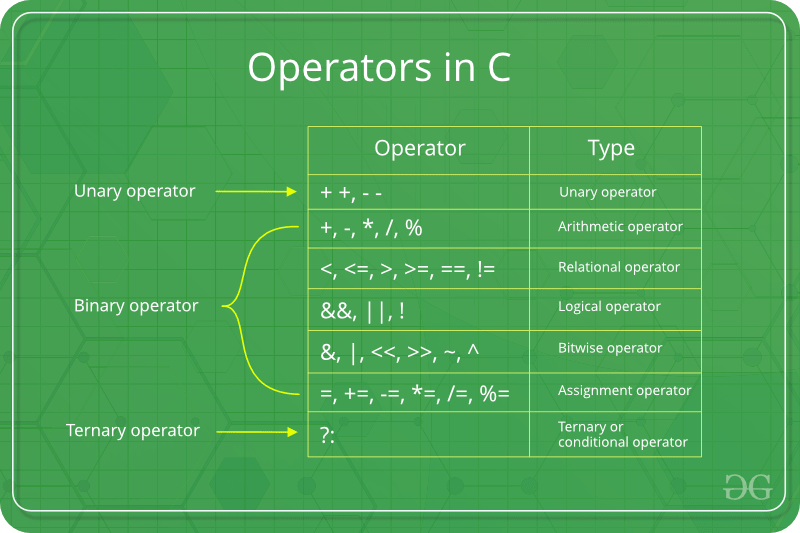Assignment operators are used to assigning value to a variable. The left side operand of the assignment operator is a variable and right side operand of the assignment operator is a value. The value on the right side must be of the same data-type of the variable on the left side otherwise the compiler will raise an error. Different types of assignment operators are shown below:

• “=” : This is the simplest assignment operator. This operator is used to assign the value on the right to the variable on the left. For example: a = 10; b = 20; ch = 'y';• C-Operators
• cpp-operator

Please write us at contrib[email protected] to report any issue with the above content

## Improve your Coding Skills with Practice## Assignment Operator in C

Using assignment operators, we can assign value to the variables.

Equality sign (=) is used as an assignment operator in C.

Here, value 5 has assigned to the variable var.

Here, value of a has assigned to the variable b . Now, both a and b will hold value 10 .

Basically, the value of right-side operand will be assigned to the left side operand.

## Pictorial Explanation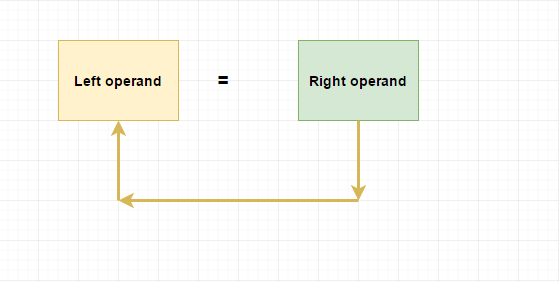## Compound assignment operators

Sample program.#### IMAGES

1. C Programming Tutorial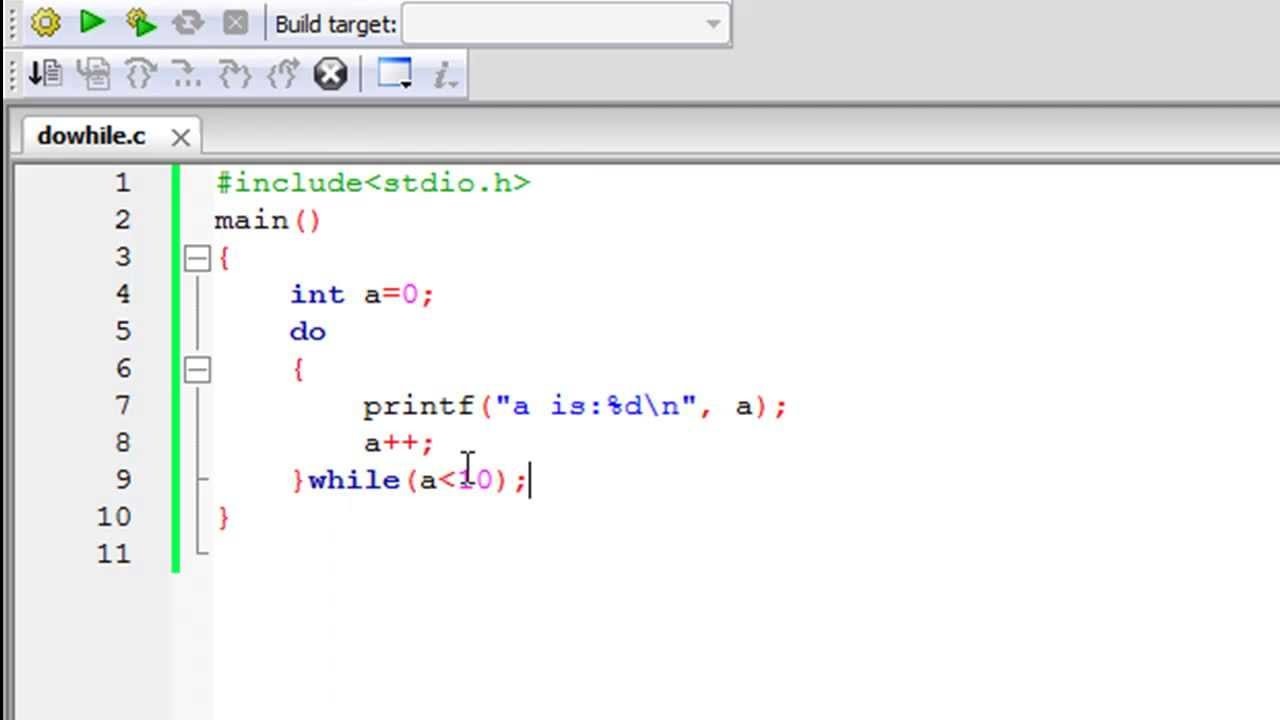2. Lecture 12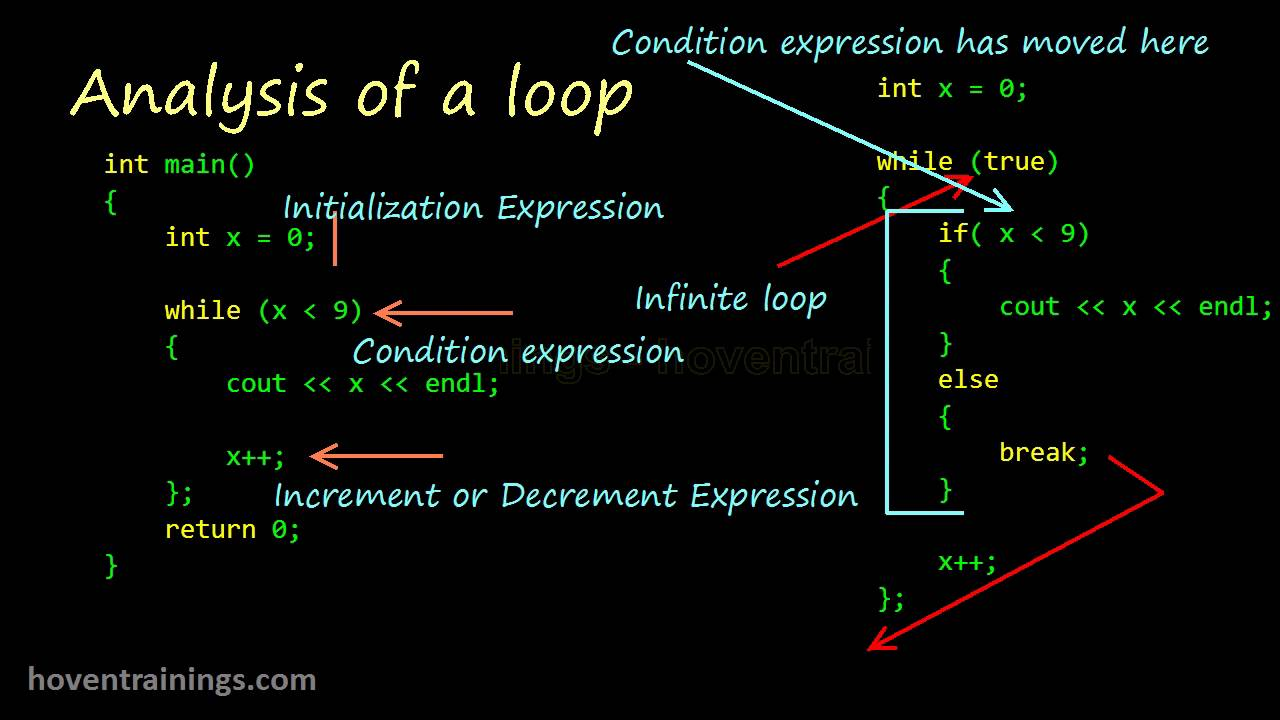3. While Loop in C/C++ Programming Example PPT4. C# While Loop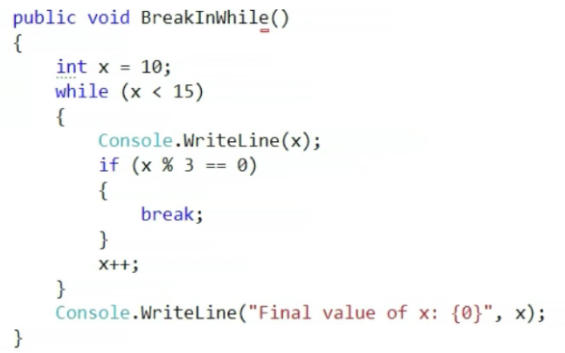5. 22-C Programming (while, do while, nested loops, arrays)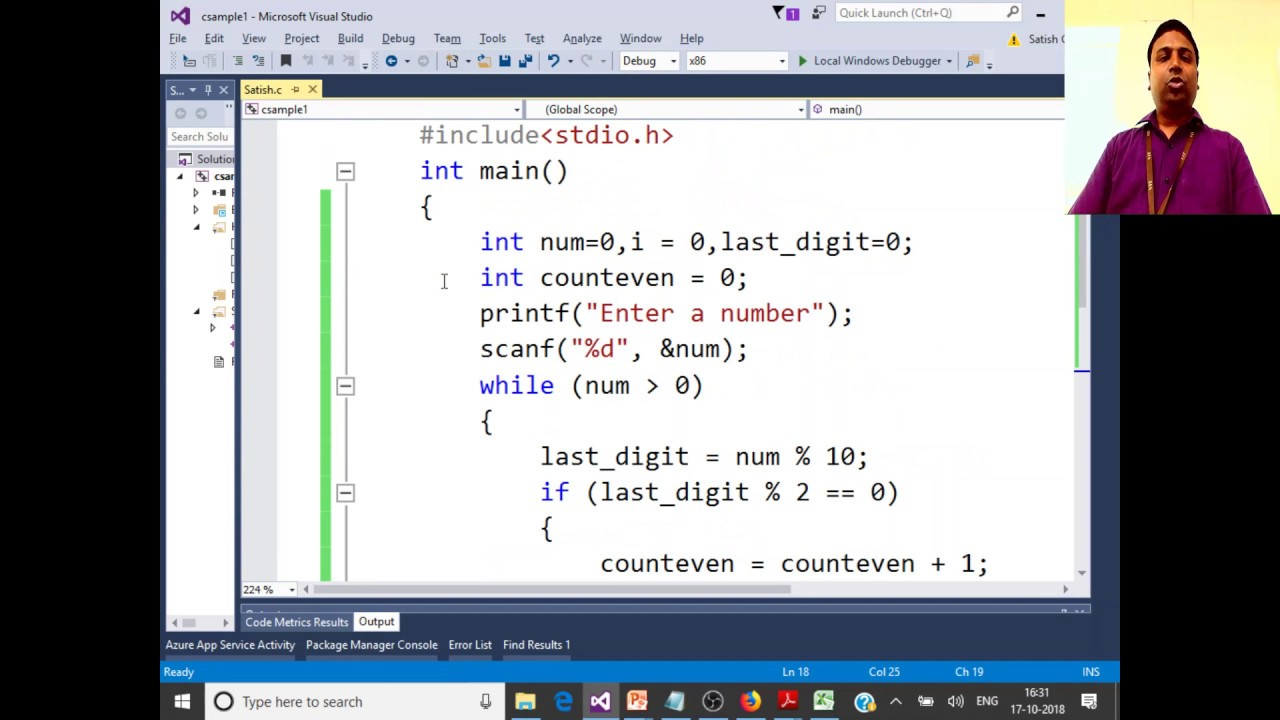6. while Loop in C Programming with example (hindi) || while statement in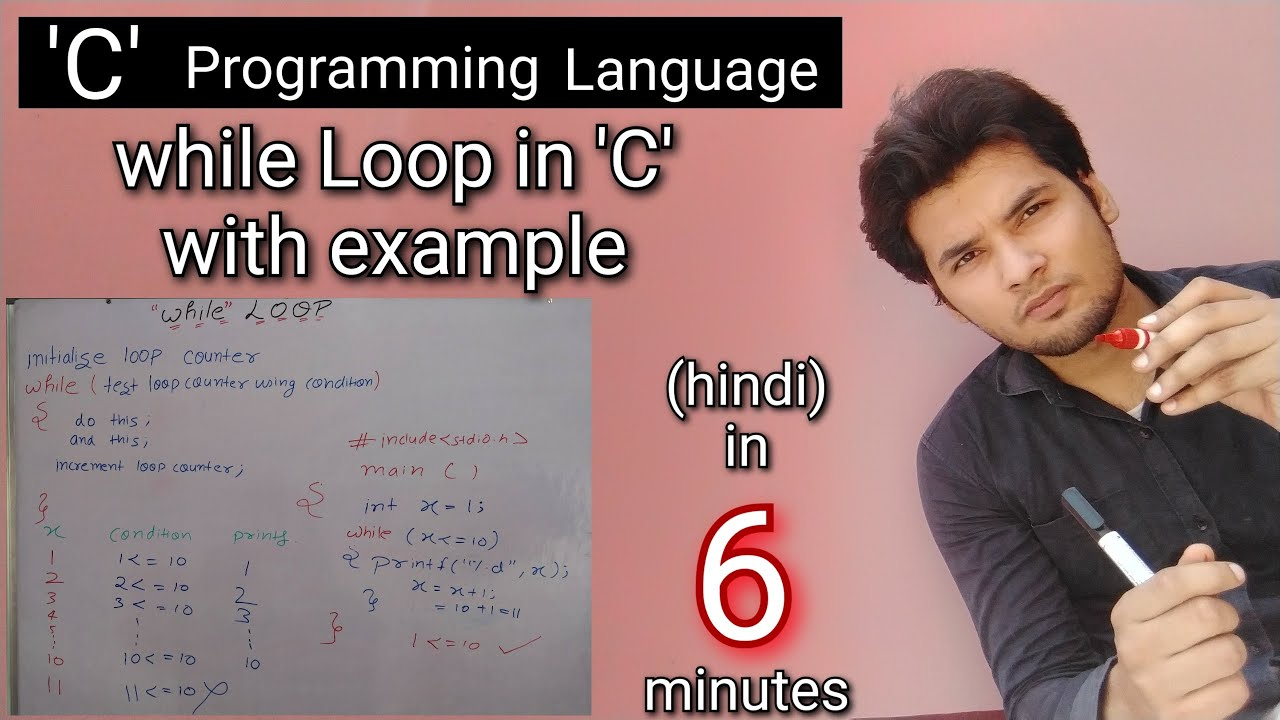#### VIDEO

1. @halokitsch performing “Moma Said (not the Rockstar) Live performance

2. Assignment Operator in C Programming

3. You Are Learning Math Wrong

4. Methods in C#

5. The Thorofare! Yellowstone Country. Flying from Cody over Ishawooa Pass and down Pass Creek

6. data type in c# part 3 int and uint in c#

1. What Is the Abbreviation for “assignment”?

According to Purdue University’s website, the abbreviation for the word “assignment” is ASSG. This is listed as a standard abbreviation within the field of information technology.

2. What Is a Deed of Assignment?

In real property transactions, a deed of assignment is a legal document that transfers the interest of the owner of that interest to the person to whom it is assigned, the assignee. When ownership is transferred, the deed of assignment show...

3. What Is a Notice of Assignment?

A Notice of Assignment is the transfer of one’s property or rights to another individual or business. Depending on the type of assignment involved, the notice does not necessarily have to be in writing, but a contract outlining the terms of...

4. Why would you use an assignment in a condition?

It is a constant fight to enforce coding without assignments in if(), while(), and other similar statements. C/C++ are notorious for side

5. Is doing an assignment inside a condition considered a code smell?

C culture is even more accepting, with the fgetc loop idiom looking like this: int c; while((c = fgetc(stream)) != EOF) {...} But in higher

6. EXP51-J. Do not perform assignments in conditional expressions

in C, it makes a lot of sense because anything can be a boolean value in C. in

7. Why do C and C++ allow assignment in conditionals when ...

There's a handful of occasions when this is slightly more elegant in C - but mostly it's there because C's assignment is an expression, and you

8. Can we put assignment operator in if condition?

Yes you can put assignment operator (=) inside if conditional statement(C/C++) and its boolean type will be always evaluated to true since

9. Do While and While Loop C/C++ Help

As we see that in while loop, if a certain condition becomes false in its first iteration then the body of while loop not executes. But in do-

10. Assigning Variables in C Using the C 4 While Loop

Error while assigning to a variable in c++, Rescanning and assigning variable in while loop, Order of evaluation in C with assigning a

11. Assignment Operator in C

Assignment Operator in C · A = 5; // use Assignment symbol to assign 5 to the operand A · B = A; // Assign operand A to the B · B = &A; // Assign the address of

12. Assignment Operator in C: Compound, Addition, Example

This operator is essential for setting initial values of variables or changing their values during the execution of a program. How can a value be assigned in C?

13. Assignment Operators in C/C++

Assignment Operators in C/C++ ... Assignment operators are used to assigning value to a variable. The left side operand of the assignment operator

14. Assignment operator in c

Using assignment operators, we can assign value to the variables. Equality sign (=) is used as an assignment operator in C. Example. int var =